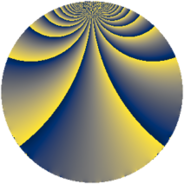# Properties

 Label 280.2.blLevel $280$ Weight $2$ Character orbit 280.bl Rep. character $\chi_{280}(221,\cdot)$ Character field $\Q(\zeta_{6})$ Dimension $64$ Newform subspaces $2$ Sturm bound $96$ Trace bound $1$

# Learn more

## Defining parameters

 Level: $$N$$ $$=$$ $$280 = 2^{3} \cdot 5 \cdot 7$$ Weight: $$k$$ $$=$$ $$2$$ Character orbit: $$[\chi]$$ $$=$$ 280.bl (of order $$6$$ and degree $$2$$) Character conductor: $$\operatorname{cond}(\chi)$$ $$=$$ $$56$$ Character field: $$\Q(\zeta_{6})$$ Newform subspaces: $$2$$ Sturm bound: $$96$$ Trace bound: $$1$$ Distinguishing $$T_p$$: $$3$$

## Dimensions

The following table gives the dimensions of various subspaces of $$M_{2}(280, [\chi])$$.

Total New Old
Modular forms 104 64 40
Cusp forms 88 64 24
Eisenstein series 16 0 16

## Trace form

 $$64q + 2q^{2} - 2q^{4} - 4q^{8} + 32q^{9} + O(q^{10})$$ $$64q + 2q^{2} - 2q^{4} - 4q^{8} + 32q^{9} - 10q^{12} + 18q^{14} - 14q^{16} - 8q^{20} - 40q^{22} - 8q^{23} - 32q^{24} + 32q^{25} - 2q^{26} - 22q^{28} + 2q^{32} - 40q^{34} + 20q^{36} - 40q^{38} - 62q^{42} + 26q^{44} + 22q^{46} - 40q^{47} + 124q^{48} + 16q^{49} + 4q^{50} + 12q^{52} - 40q^{54} - 32q^{55} + 24q^{56} - 32q^{57} + 6q^{58} + 14q^{60} - 16q^{62} - 80q^{63} + 4q^{64} + 60q^{66} + 28q^{68} + 20q^{70} - 48q^{71} - 60q^{72} + 16q^{73} - 42q^{74} - 20q^{76} - 40q^{78} + 40q^{79} - 40q^{81} - 34q^{82} - 8q^{84} - 56q^{86} + 48q^{87} - 4q^{88} + 8q^{89} - 36q^{90} + 84q^{92} - 66q^{94} - 16q^{96} - 64q^{97} - 58q^{98} + O(q^{100})$$

## Decomposition of $$S_{2}^{\mathrm{new}}(280, [\chi])$$ into newform subspaces

Label Dim. $$A$$ Field CM Traces $q$-expansion
$$a_2$$ $$a_3$$ $$a_5$$ $$a_7$$
280.2.bl.a $$4$$ $$2.236$$ $$\Q(\zeta_{12})$$ None $$-2$$ $$0$$ $$0$$ $$-8$$ $$q+(-\zeta_{12}-\zeta_{12}^{2}+\zeta_{12}^{3})q^{2}+2\zeta_{12}q^{4}+\cdots$$
280.2.bl.b $$60$$ $$2.236$$ None $$4$$ $$0$$ $$0$$ $$8$$

## Decomposition of $$S_{2}^{\mathrm{old}}(280, [\chi])$$ into lower level spaces

$$S_{2}^{\mathrm{old}}(280, [\chi]) \cong$$ $$S_{2}^{\mathrm{new}}(56, [\chi])$$$$^{\oplus 2}$$